# 引入

1. 两个节点连接（保证不出现环）
2. 两个节点断开
3. 求任意两个节点之间的区间和

# 样例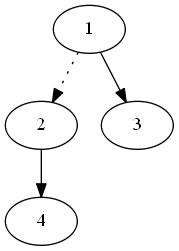# 操作

## 操作一（一切的基础）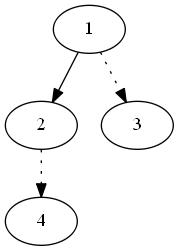## 操作二(Splay=神奇的相对位置)

1、1->2
2、4
3、3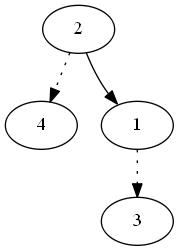## 操作三(make_root=让整个树换个方向吧）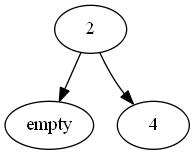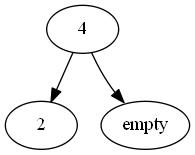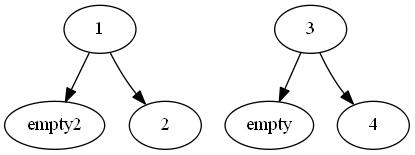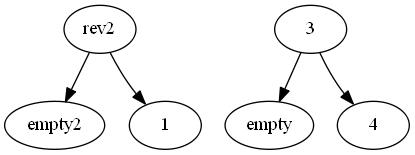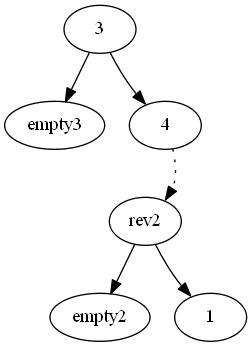# 代码

#include <cstdio>
#include <cstring>
#include <algorithm>
#include <iostream>
using namespace std;
const int MAXN = 10000;
int ch[MAXN+10], Fa[MAXN+10], rev[MAXN+10], que[MAXN+10];
bool isroot(int u){
if(!Fa[u]) return 1;
return ch[Fa[u]] != u && ch[Fa[u]] != u;
}
void Rotate(int u){
bool d = ch[Fa[u]] == u;
int x = Fa[u], y = Fa[x];
Fa[u] = y;
if(!isroot(x)) ch[y][ch[y] == x] = u;
ch[x][d] = ch[u][!d]; Fa[ch[u][!d]] = x;
ch[u][!d] = x; Fa[x] = u;
}
void push_down(int u){
if(rev[u]){
rev[ch[u]]^=1;
swap(ch[ch[u]], ch[ch[u]]);
rev[ch[u]]^=1;
swap(ch[ch[u]], ch[ch[u]]);
rev[u] ^= 1;
}
}
void Splay(int u){
int top = 0;
que[++top] = u;
for(int i=u;!isroot(i);i=Fa[i])
que[++top] = Fa[i];
top++;
while(--top) push_down(que[top]);
while(!isroot(u)){
int x = Fa[u], y = Fa[x];
if(!isroot(x)){
if((ch[y] == x) ^ (ch[x] == u)) Rotate(x);
else Rotate(u);
}
Rotate(u);
}
}
void Access(int u){
int t = 0;
while(u){
Splay(u);
ch[u] = t;
t = u;
u = Fa[u];
}
}
void reset_root(int u){
Access(u);
Splay(u);
rev[u] ^= 1;
swap(ch[u], ch[u]);
}
reset_root(x);
Fa[x] = y;
Splay(x);
}
void Cut(int x, int y){
reset_root(x);
Access(y);
Splay(y);
ch[y] = Fa[x] = 0;
}

# 感谢©️2020 CSDN 皮肤主题: 大白 设计师: CSDN官方博客点击重新获取扫码支付1.余额是钱包充值的虚拟货币，按照1:1的比例进行支付金额的抵扣。
2.余额无法直接购买下载，可以购买VIP、C币套餐、付费专栏及课程。余额充值